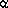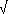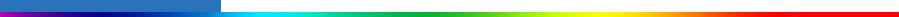Working Group I: The Scientific BasisGet Javascript Other reports in this collection

6.3.4 Total Well-Mixed Greenhouse Gas Forcing Estimate

 Table 6.2: Simplified expressions for calculation of radiative forcing due to CO2, CH4, N2O, and halocarbons. The first row for CO2 lists an expression with a form similar to IPCC (1990) but with newer values of the constants. The second row for CO2 is a more complete and updated expression similar in form to that of Shi (1992). The third row expression for CO2 is from WMO (1999), based in turn on Hansen et al. (1988).Trace gas Simplified expression Radiative forcing,F (Wm-2) ConstantsCO2F =ln(C/C0)= 5.35F=ln(C/C0) + ß (C -C0)= 4.841, ß = 0.0906F=(g(C)�g(C0)) where g(C)= ln(1+1.2C+0.005C2 +1.4 x 10-6C3)= 3.35CH4F=(M �M0) � (f(M,N0) � f(M0,N0))= 0.036N2OF=(N �N0) � (f(M0,N) � f(M0,N0))= 0.12CFC-11aF=(X � X0)= 0.25CFC-12F=(X � X0)= 0.32f(M,N) = 0.47 ln[1+2.01x10-5 (MN)0.75+5.31x10-15 M(MN)1.52] C is CO2 in ppm M is CH4 in ppb N is N2O in ppb X is CFC in ppb The constant in the simplified expression for CO2 for the first row is based on radiative transfer calculations with three-dimensional climatological meteorological input data (Myhre et al., 1998b). For the second and third rows, constants are derived with radiative transfer calculations using one-dimensional global average meteorological input data from Shi (1992) and Hansen et al. (1988), respectively. The subscript 0 denotes the unperturbed concentration. a The same expression is used for all CFCs and CFC replacements, but with different values for(i.e., the radiative efficiencies in Table 6.7).